### Home > AC > Chapter 16 > Lesson 16.9.2.2 > Problem9-81

9-81.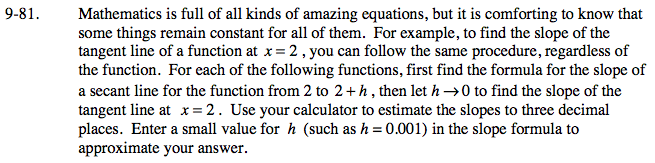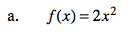$m=\frac{2(2+h)^{2}-f(2)}{h}$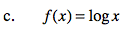$m=\frac{\log (2+h)-\log(2)}{h}$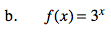$m=\frac{3^{(2+h)}-3^{2}}{h}$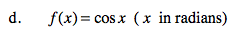$m=\frac{\cos(2+h)-\cos(2)}{h}$

Use the eTool below for your estimates.
Click the link at right for the full version of the eTool: PCT 9-81 HW eTool